# Kerala SSLC Maths Previous Year Question Paper March 2019 English Medium

Students can Download Kerala SSLC Maths Previous Year Question Paper March 2019 English Medium, Kerala SSLC Maths Model Question Papers helps you to revise the complete Kerala State Syllabus and score more marks in your examinations.

## Kerala SSLC Maths Previous Year Question Paper March 2019 English Medium

Time: 2½ Hours
Total Score: 80 Marks

Instructions

• Give explanations wherever necessary.
• First 15 minutes is Cool-off time. You may use the time to read the questions and plan your answers.
• No need to simplify irrationals like √2, √3, π etc., using approximations unless you are asked to do so.

Answer any three questions from 1 to 4. Each question carries 2 scores. (3 × 2 = 6)

Question 1.
In the figure, O is the centre of the circle. ∠AOC = 80°
a) What is the measure of ∠ABC?
b) What is the measure of ∠ADC?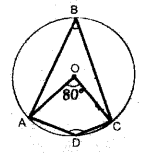a) $$\angle \mathrm{ABC}=\frac{1}{2} \times \angle \mathrm{AOC}=\frac{1}{2} \times 80=40^{\circ}$$
b) ∠ADC = 180 – ∠ABC = 180 – 40 = 140°

Question 2.
a) Write the first integer term of the arithmetic sequence $$\frac{1}{7}, \frac{2}{7}, \frac{3}{7} \ldots$$
b) What is the sum of the first 7 terms of this se-quence?
a) First integer term is $$\left(\frac{7}{7}\right)=1$$
b) Sum of first 7 terms = $$\frac { 1 }{ 7 }$$ (1 + 2 + 3 + ….. + 7)
= $$=\frac{1}{7} \times \frac{7 \times 8}{2}=4$$Question 3.
a) If C(-1, k) is a point on the line passing through the points A(2, 4) and B(4, 8). Which number is k?
b) What is the relation between the x coordinate and the y coordinate of any point on this line?
a) Since C is on the line AC,
slope of AB = slope of BC
\begin{aligned} \frac{8-4}{4-2} &=\frac{8-k}{4–1} \\ \frac{4}{2} &=\frac{8-k}{5} \end{aligned}
⇒ 4 × 5 = 2 (8 – k) = 20
⇒ 20 = 16 – 2k
⇒ 2k = 16 – 20 = -4
⇒ k = -2
b) Let (x, y) be a point on the line
\begin{aligned} &\frac{y-4}{x-2}=\frac{8-4}{4-2}\\ &\frac{y-4}{x-2}=\frac{4}{2} \end{aligned}
⇒ 2 (y – 4) = 4(x – 2)
⇒ 2y – 8 = 4x – 8
⇒ 4x – 2y – 8 + 8 = 0
⇒ 4x – 2y = 0
⇒ 2x – y = 0
⇒ y = 2x

Question 4.
a) Find P(1) if P(x) = x2 + 2x + 5
b) If (x – 1) is a factor of x2 + 2x + k, What number is k?
a) P(1) = 12 + 2 × 1 + 5 = 1 + 2 + 5 = 8
b) Since x – 1 is a factor
P(1) = 0
⇒ 12 + 2 × 1 + k = 0
⇒ 1 + 2 + k = 0
⇒ k = -3

Answer any five questions from 5 to 11. Each question carries 3 scores. (5 × 3 = 15)

Question 5.
a) What is the remainder on dividing the terms of the arithmetic sequence 100, 107, 114.. by 7?
b) Write the sequence of all three digit numbers. Which leaves remainder 3 on division by 7? Which is the last term of this sequence?
a) 100 = 7 × 14 + 2
107 = 7 × 15 + 2
Remainder on dividing the terms by 7 is 2
b) x1 = 101, x2 = 108, ……
Sequence : 101, 108, 115 ….
xn = dn + (f – d) = 7n + 94
To find largest 3 digit terms
7n + 94 < 1000
7n < 906
n < $$\frac { 906 }{ 7 }$$ = 129.4 = 129
x129 = 7 × 129 + 94 = 997Question 6.
AB is the diameter of the. circle. D is a point on the circle. ∠ACB + ∠ADB + ∠AEB = 270°. The measure of one among ∠ACB, ∠ADB, ∠AEB is 110°. Write the measures of ∠ADB, ∠ACB, and ∠AEB.Since AB is the diameter, ∠ADC = 90°
∠ACB is obtuse.
∴ ∠ACB = 110°
∴ ∠AEB = 270 – (90 + 110) = 270 – 200 = 70°
∴ ∠ADB = 90°, ∠ACB = 110°, ∠AEB = 70°

Question 7.
If x is a natural number
a) What number is to be added to x2 + 6x to get a perfect square?
b) If x2 + ax + 16 is a perfect square which number is ‘a’?
c) If x2 + ax + b is a perfect square prove that a2 = 4b.
a) x2 + 6x = x2 + 2 × x × 3
Add 32 to it we get
x2 + 2x × 3 + 9 = (x + 3)2
b) x2 + ax + 16 = x2 + ax + 42
ax = 2 × x × 4 = 8x
∴ a = 8 or a = -8
c) x2 + ax + b is a perfect square
$$x^{2}+2 \times x \times \frac{a}{2}+\left(\frac{a}{2}\right)^{2}$$ is a perfect square
\begin{aligned} &b=\left(\frac{a}{2}\right)^{2}\\ &b=\frac{a^{2}}{4}, a^{2}=4 b \end{aligned}

Question 8.
In the figure ∠B = 90°, ∠C = 44°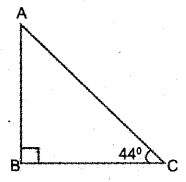a) What is the measure of ∠A?
b) Which among the following is tan 44°?
$$\left(\frac{\mathrm{AB}}{\mathrm{BC}}, \frac{\mathrm{AB}}{\mathrm{AC}}, \frac{\mathrm{BC}}{\mathrm{AB}}, \frac{\mathrm{BC}}{\mathrm{AC}}\right)$$
c) Prove that tan 44° × tan 46° = 1.
a) ∠A = 90 – 44 = 46°
b) $$\frac{A B}{B C}$$
c) $$\tan 44^{\circ} \times \tan 46^{\circ}=\frac{\mathrm{AB}}{\mathrm{BC}} \times \frac{\mathrm{BC}}{\mathrm{AB}}=1$$

Question 9.
Draw a circle of radius 3 centimetres. Mark a point P at a distance 6 centimetres from the centre of the circle. Draw tangents from P to the circle.

• Draw the circle having radius 3 cm, mark the centre C, and point P at the distance 6 cm from the centre.
• Draw perpendicular bisector of CP and mark midpoint of CP as O.
• Draw a circle with centre O and radius OP. The circle cut the first circle at A and B
• PA and PB are the tangents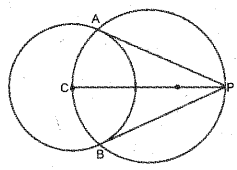Question 10.
a) Find the coordinates of the point on the x-axis, which is at a distance 4 units from (3, 4).
b) Find the coordinates of the points on the x-axis at a distance 5 units from (3, 4).
a) The point on x axis at the distance 4 cut from (3, 4) is (3, 0)
b) Let P (x, 0) be the point
(x – 3)2 + (0 – 4)2 = 52
⇒ (x – 3)2 = 25 – 16
⇒ (x – 3)2 = 9
⇒ x – 3 = 3, -3
If x – 3 = 3, x = 6
If x – 3 = -3, x = 0
Points : (0, 0), (6, 0)

Question 11.
The given figure is the lateral face of a square pyramid. AB = AC = 25 centimetres and BD = DC = 15 centimetres.
a) What is the length of its base edge?
b) Find the lateral surface area of the pyramid.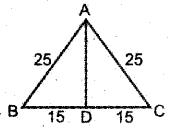a) a = 30 cm
b) AD2 + CD2 = AC2
AD2 = 625 – 225 = 400
Latral face area = 2al = 2 × 30 × 20 = 1200 cm2

Answer any 7 questions from 12 to 21. Each question carries 4 scores. (7 × 4 = 28)

Question 12.
In triangle ABC, ∠A = 30°, ∠B = 80°, circumradius of the triangle is 4 centimetres. draw the triangle. Measure and write the length of its smallest side.

• Draw the circle of radius 4 cm and a radius OA.
• Mark a point Bon the circle such that ∠COB = 60° and draw OB.
• Mark a point C on the circle such that ∠AOC = 160°
• Join AB, BC and AC, and complete ∆ABC.

Question 13.
Find the following sums:
a) 1 + 2 + 3 + ……………. + 100
b) 1 + 3 + 5 + ………….. + 99
c) 2 + 4 + 6 + ………….. + 100
d) 3 + 7 + 11 + ……………. + 199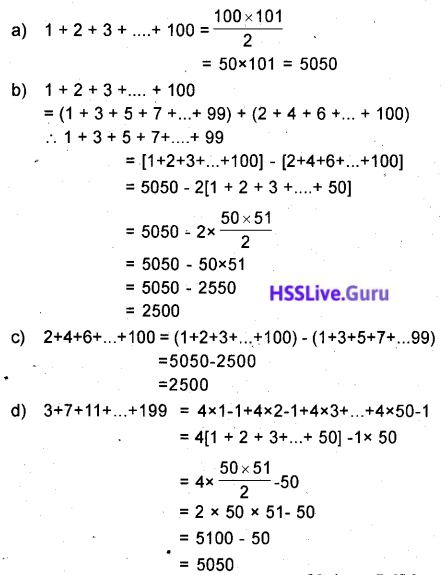Question 14.
A box contains some green and blue balls. 7 red balls are put into it. Now the probability of getting a
red ball from the box is $$\frac{7}{24}$$ and that of a blue ball is $$\frac{1}{6}$$
a) How many balls are there in the box?
b) How many of them are blue?
c) What is the probability of getting a green ball from the box?
a) Total number of balls = 24
b) Let x be the number of blue balls
$$\frac{x}{24}=\frac{1}{3}$$
⇒ 3x = 24
⇒ x = 8
c) Number of green balls = 24 – (7 + 8) = 24 – 15 = 9
Probability of. getting green ball $$=\frac{9}{24}=\frac{3}{8}$$Question 15.
The land is acquired for road widening from a square ground, as shown in the figure. The width of the acquired land is 2 metres. Area of the remaining, ground is 440 square metres.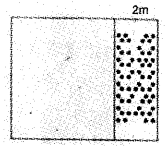a) What is the shape of the remaining ground?
b) What is the length of the remaining ground?
a) Rectangle
b) x (x – 2) = 440
⇒ x2 – 2x = 440
⇒ x2 – 2x + 1 = 441
⇒ (x – 1)2 = 212
⇒ x – 1 = 21
⇒ x = 1 + 21 = 22
Length 22m, width 20m

Question 16.
In the figure, P is the centre of the circle. A, B and D are points on the circle. ∠P = 90°, AD = 5 centimetres.a) What is the measure of ∠A?
b) What is the area of triangle APD?
c) Find the area of the parallelogram ABCD
a) AP = PD, ∠A = 45°
b) Area of APD = $$\frac { 1 }{ 2 }$$ × AP × PD
\begin{aligned} &=\frac{1}{2} \times \frac{5}{\sqrt{2}} \times \frac{5}{\sqrt{2}}\\ &=\frac{25}{4} \end{aligned}
= 6.25 cm2
c) Area of ABCD = AB × PD
$$=2 \times \frac{5}{\sqrt{2}} \times \frac{5}{\sqrt{2}}$$
= 25 cm2

Question 17.
a) Draw the coordinate axes and mark the points A(1, 1), B(7, 1)
b) Draw an isosceles right triangle ABC with AB as hypotenuse.
c) Write the coordinates of C.
a) A(1, 1) and B(7, 1)
b) The midpoint of AB is E (4, 1)
AE = 3 unit
Move 3 unit up from E and mark C (4, 4).
ABC will be an isosceles right triangle.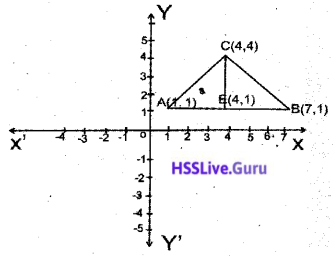c) Coordinates of C = (4, 1 + 3) = (4, 4)Question 18.
In the figure chord, BC is extended to P. Tangent from P to the circle is PA. AQ is the bisector of ∠BACa) Write one pair of equal angles from the figure.
b) If ∠PAC = x and ∠PCA = y prove that ∠BAC = y – x
c) Prove that ∠PAQ = $$\frac{y+x}{2}$$
a) ∠ABC = ∠CAP
b) ∠B = ∠PAC = x
∠B = ∠BAC = ∠ACP = y
x + ∠BAC = y
∠BAC = y – x
c) ∠PAQ = ∠PAC + ∠CAQQuestion 19.
If x – 1 is a factor of the second degree polynomial P(x) = ax2 + bx + c and P(0) = -5.
a) What is the value of c?
b) Prove that a + b = 5.
c) Write a second degree polynomial whose one factor is x = 1.
a) P(0) = -5 , c = -5
b) P(1) = 0, a + b + c = 0
a + b – 5 = 0
a + b = 5
c) If x – 1 is a factor, sum of the coefficients willbe zero.
P(x) = 3x2 + 2x – 5 = 0

Question 20.
A circular sheet of paper is divided into two sectors. The central angle of one of them is 160°.
a) What is the centre of the remaining sector?
b) These sectors are bent into cones of maximum volume. If the radius of the small cone is 8 centimetres, what is the radius of the other?
c) What is the slant height of the cones?
a) Central angle of second sectoral plate = 360 – 160 = 200°
l × 160 = 360 × 8
$$\ell=\frac{360 \times 8}{160}$$ = 18 cm
Radii of sectors are equal .
\begin{aligned} &\frac{r}{18}=\frac{200}{360}\\ &\begin{aligned} r &=18 \times \frac{200}{360} \\ &=10 \mathrm{cm} \end{aligned} \end{aligned}
Radius of the big cone = 10 cm
c) 18 cm (Equal to radius of the circle)Question 21.
Equation of the line AB is 3x – 2y = 6. P is a point on the line. The line intersects the y-axis at A and the x-axis at B.
a) What is the x coordinate of A?
b) What is the length of OA?
c) What is the length of OB?
d) The x coordinate and the y coordinate of P are the same. Find the coordinates of P.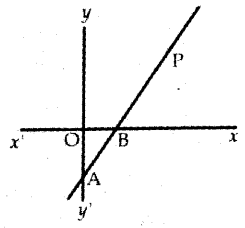a) x coordinate of A = 0
b) When x = 0,
3x – 2y = 6, y = -3
Coordinates of A (0, -3)
OA = 3 unit
c) y coordinate of B is 0
3x – 2 × 0 = 6, x = 2
Coordinates of B (2, 0)
OB = 2 unit
d) Since coordinates fo P are (x, x)
3x – 2x = 6
x = 6
P (6, 6)

Answer any five questions from 22 to 28. Each question carries 5 score. (5 × 5 = 25)

Question 22.
If the terms of the arithmetic sequence $$\frac{2}{9}, \frac{3}{9}, \frac{4}{9}, \frac{5}{9} .$$ are represented as x1, x2, x3, ….. then
a) x1 + x2 + x3 = ______
b) x4 + x5 + x6 = _______
c) Find the sum of first 9 terms.
d) What is the sum of first 200 terms?d) Sum of 300 terms = 1 + 2 + 3 + ….. + 100
$$=\frac{100 \times 101}{2}$$
= 5050

Question 23.
Draw a rectangle of area 12 square centimetres. Draw a square having the same area.

1. Draw rectangle ABCD of side AB = 6 cm, BC = 2 cm.
2. Produce AB to E such that BC = BE
3. Mark the midpoint of AB as O
4. Draw a semicircle with O as the centre and OE as the radius.
5. BC, when produced meet the semicircle at G.
6. Draw a square witQuestion 24.
A boy standing at one bank of a river sees the top of a tree on the other bank directly opposite to the boy pt an elevation of 60°. Stepping 40 metres back, he sees the top at an elevation of 30°.
a) Draw a rough figure and find the height of the tree
b) What is the width of the river?
a) PC = 40 m
∠BPC = 30°
∠BCA = 60°In ΔPCB, ∠PCB = 120°
∠PBC = 30°
ΔPCB is an isoscles triangle
BC = PC = 40 cm
Consider ΔCBA
This is a 30° – 60° – 90° triangle side opposite to 30° in ΔCAB is = 20 m
AB = 2 × \frac{40}{\sqrt{3}} = 20√3 m
b) Width of the river is 20 m

Question 25.
Circle with centre O touches the sides of the triangle at P, Q and R, AB = AC, AQ = 4 centimetres and CQ = 6 centimetres.a) What is the length of CP?
b) Find the perimeter and the area of the triangle.
c) What is the radius of the circle?
a) CP = CQ = 6 cm
b) AC = AQ + QC = 4 + 6 = 10 cm
AB = AC = 10 cm
AR = AQ = 4 cm
BR = AB – AR = 10 – 4 = 6 cm
BP = BR = 6 cm
BC = BP + PC = 6 + 6 = 12 cm
Perimeter of ∆ABC = AB + BC + AC = 10 + 12 + 10 = 32 cm
Area of ∆ABC = $$\frac { 1 }{ 2 }$$ × BC × AP
= $$\frac { 1 }{ 2 }$$ × 12 × 8
= 48 cm2 $$[\mathrm{AP}=\sqrt{\mathrm{AB}^{2}-\mathrm{BP}^{2}}]$$
c) $$r=\frac{A}{S}=\frac{48}{16}=3 \mathrm{cm}$$Question 26.
The radius of a cylinder is equal to its height. If the radius is taken as r, the volume of the cylinder is πr2 × r = πr3. Like this find the volumes of the solids, with the following measures.

 Solids Measures Volume Cone Radius = height = r Hemisphere Radius = r Sphere Radius = r

a) What is the ratio of the volumes of the cone, hemisphere, cylinder and the sphere?
b) A solid metal sphere of radius 6 centimetres is melted and recast into solid cones of radius 6 centimetres and height 6 centimetres. Find the number of cones.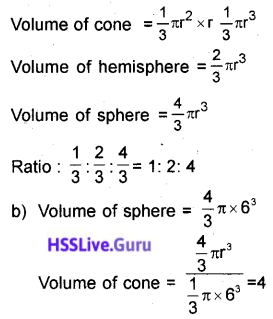Question 27.
C is the centre of the circle passing through the origin. Circle cuts the y-axis at A(0, 4) and the x-axis at B(4, 0)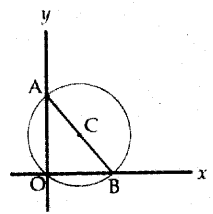a) Write coordinates of C
b) Write the equation of the circle.
c) (0, 0) is the point on the circle. There is one more point on the circle with x and y coordinates equal. Which is that point?
a) $$C\left(\frac{0+4}{2}, \frac{4+0}{2}\right)=C(2,2)$$
b) Radius $$=\frac{A B}{2}=\frac{4 \sqrt{2}}{2}=2 \sqrt{2}$$ Unit [1 : 1 : √2 ]
Equation = (x – 2)2 + (y – 2)2 = (2√2)2
= x2 – 4x + 4 + y2 – 4y + 4 = 8
= x2 + y2 – 4x – 4y = 0
c) If x = y
x2 + x2 – 4x – 4x = 0
2(x2 – 4x) = 0
x2 – 4x = 0
x(x – 4) = 0
x = 0, x = 4
The required point is (4, 4)

Question 28.
The table below shows the number of children in a class, sorted according to their heights.

 Height (Centimetres) Number of Children 130 – 140 7 140 – 150 9 150 – 160 10 160 – 170 10 170 – 180 9

If the students are directed to stand in a line according to the order of their heights starting from the smallest, then
a) The height of the child at what position is taken as the median?
b) What is the assumed height of the child in the 17th position?
c) Find the median height.
n = 7 + 9 + 10 + 10 + 9 = 45 (odd)

 Height (cm) No. of children Less than 140 7 Less than 150 16 Less than 160 26 Less than 170 36 Up to 180 45

a) $$\frac{45+1}{2}$$ = 23rd chil’s height comes in the middle.
the median when 10 cm height in divided into 10 children, each one’s share is $$\frac{10}{10}=1$$
Height of 17th child = 150 + $$\frac { 1 }{ 2 }$$ = 150.5
b) Take 150.5 as the fist term and 1 as the common difference 7th term is the height of 23rd child.
c) Median = 150.5 + 6 × 1 = 150.5 + 6 = 156.5 cm.

Read the following. Understand the Mathemati¬cal concepts in it and answer the questions. (6 × 1 = 6)

Question 29.
The remainders obtained on dividing the powers of two by 7 have an interesting property. We can understand it from the table given below.If the powers are 1, 4, 7, … the remainder is 2
If the powers are 3, 6, 9,.. the remainder is 1
a) What is the remainder on dividing 28 by 7?
b) Write the sequence of powers of 2 leaving remainder 1 on division by 7.
c) Check whether 2019 is a term f the arithmetic sequence 3, 6, 9,…
d) What is the remainder on dividing 22019 by 7?
e) Write the algebraic form of the arithmetic sequence 1, 4, 7 …
f) Write the algebraic form of the sequence 21, 24, 27, ……. (powers of two leaving remainder 2 on division by 7).